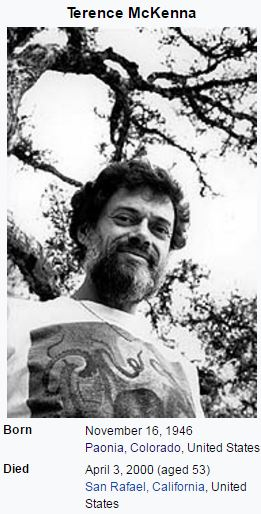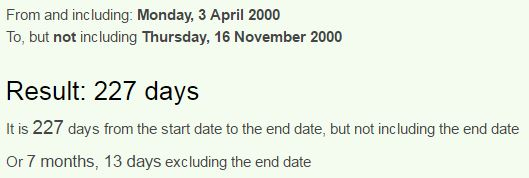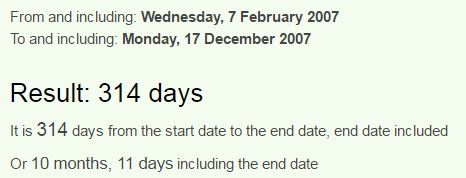CipherApr 18, 2017

Terence McKenna murder by the numbers... 227/318

This is gonna be a short post on the murder of Terence McKenna... I honestly think there's no chance that he died from a "rare form of brain cancer"Terence McKenna died on April 3rd, 93rd day and a date coming 227 days before his birthdayAlso note 7 months 13 days... a lot like 713 or the reflection 317 (66th prime number)
Dennis McKenna = 4+5+40+40+9+90 + 30+3+10+5+40+40+1 = 317 (Jewish)Note that his brother is born on the first day of ancient Roman Holiday called Saturnalia, this is very interesting because Terence died on 93rd day of the year, Saturn = 93 (English Ordinal), Terence =  119 (Reverse Ordinal), Saturn = 45+1+20+21+18+14 = 119 (Francis Bacon)
From the birthday of his brother in 1999 to his death is 3 months and 18 days, connecting back to Pi (22/7 = 3.14, 7/22 = 0.318....)McKenna died on a date with 27 numerology (4+3+20+00 = 27) and this is very interesting considering his Library burned down 7 years after his death on February 7th, written 2/7 in the USNote that this came 314 days before birthday of his brotherFebruary 7th is the 38th day of the year
Terence = 7+4+9+4+4+6+4 = 38 (Reverse Reduction)
McKenna = 5+6+7+4+4+4+8 = 38 (Reverse Reduction)
This library fire took place 1 month and 28 days before anniversary of his death, connecting to his last name...McKenna = 14+24+16+22+13+13+26 = 128 (Reverse Ordinal)